Related Articles
Sorting of Strings in Julia
• Last Updated : 05 Sep, 2020

Sorting of strings in Julia means that now we are sorting the characters of the string. In Julia, this can easily be achieved with the basic logic of getting each character of the string into a separate array and sorting the array. Lastly joining the array together to get the sorted array.

1. Fetching each character of the string and putting it into a separate array.
2. Applying the sorting algorithms or different sorting functions on the fetched characters.
3. Joining the array of characters and getting the sorted string as output.

#### Creating an Array of String Characters

To create an array of the characters present in the string, Julia provides a pre-defined function collect(). This function returns the elements in the form of array in our case which are characters here if the string is passed into this function.

Example

## Julia

 `# Defining a String ` `str1 ``=` `string(``"julia"``) ` ` `  `# Using the collect function ` `# to fetch each character of the string ` `a ``=` `collect(str1)`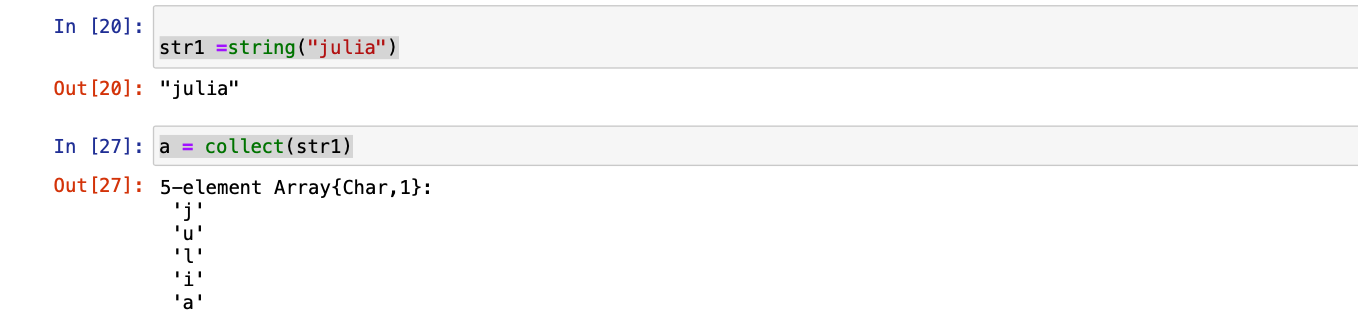#### Sorting of Strings

Julia provides various methods and pre-defined functions for the sorting of strings. These methods take an array of characters as an argument and return the sorted array.

#### Method 1: Using sort() function

This sorts the elements of the array and can be used to sort an array of integers, characters, etc. This function sorts algorithm with time complexity of O(N log N).Here, in this function string str1 is passed as argument.

## Julia

 `str1 ``=` `string(``"julia"``) ` ` `  `a ``=` `collect(str1) ` ` `  `# Applying the sort function ` `b ``=` `sort(a)`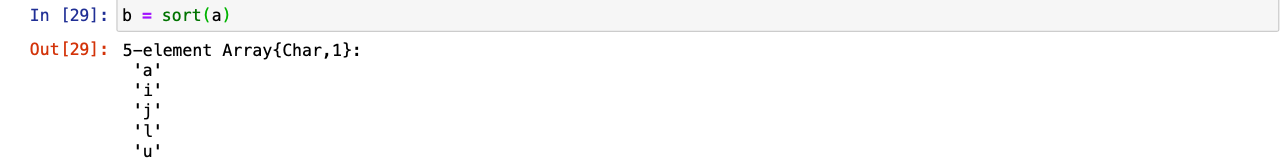#### Joining Array elements to make a String

To sort the string, the sorted array elements need to be joined back together to form a string. Julia provides a pre-defined function join() that joins the character array and forms a substring of each character in it. The sorted characters stored in b is now passed as argument in join() function.

## Julia

 `# Joining the sorted characters ` `join(b)`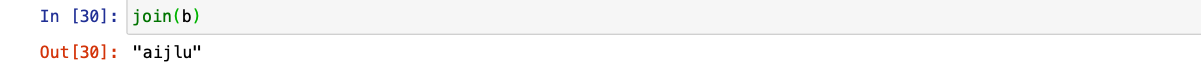#### Splitting Strings into Substrings

Splitting of Strings is done to split the array into multiple substrings which are sorted individually with the help of arrays and are joined back once sorted. Julia provides a pre-defined function split() that works same as collect() function means it returns each character of the string remember as substrings.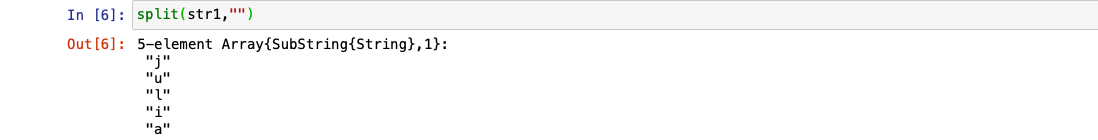#### Method 2:  Using sort(fetchedsubstrings ,alg=) function

It works in a similar manner as sort function but with a better time complexity. The time complexity equals to the time complexity of the algorithm used and this algorithm is passed as argument in the above function

Using Quicksort (Best case Time Compexity=O(n log n)) algorithm

Here the returned array from collect or split is passed as an argument in the function and the sorting algorithm. Then joining the sorted substrings using the join() function.

## Julia

 `str1 ``=``string(``"julia"``)  ` ` `  `a ``=` `collect(str1) ` ` `  `# using Quicksort algorithm ` `sort(a, alg ``=` `QuickSort) ` ` `  `join(b)`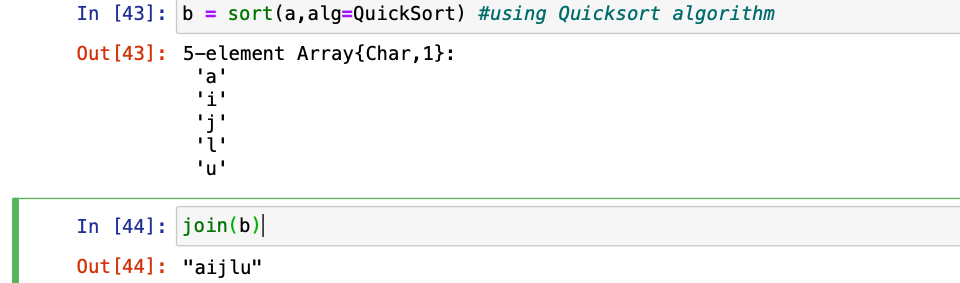#### Using Insertionsort (Best case Time Complexity =O(n)) Algorithm

Here the returned array from the collect or split function is passed as an argument in the function along with the algorithm to be used. Then joining the sorted substrings using the join() function.

## Julia

 `str1 ``=` `string(``"julia"``)  ` ` `  `a ``=` `collect(str1) ` ` `  `# using Insertionsort algorithm ` `b ``=` `sort(a, alg ``=` `InsertionSort) ` ` `  `join(b)`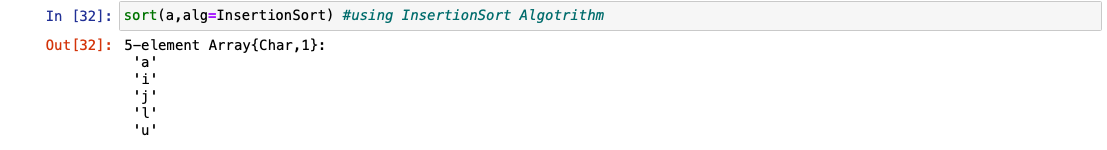#### Method 3: Using sortperm(fetchedsubstrings) Function

This function returns a list of indices that one can use on the collection to produce a sorted collection by simply passing the fetched substrings as an argument.

Here the fetched substrings will be passed as an argument in sortperm(a) function.

## Julia

 `str1 ``=` `string(``"julia"``)  ` ` `  `a ``=` `collect(str1) ` ` `  `# using sortperm() function ` `# returns the sorted indexes  ` `# of each substring and storing in 'k' ` `k ``=` `sortperm(a) `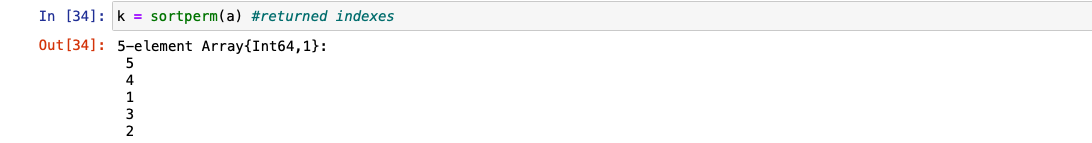Now we will traverse the array of substrings using the for loop and passing the indexes stored in k in the array of substrings.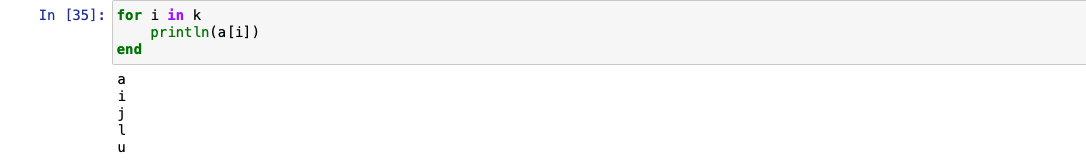#### Method  4: Using sort(fetchedsubstrings, rev = true) Function

This function will sort the array into descending order as we have changed the default value of rev to true which is actually false. Here, after sorting in descending order we will join the sorted substrings using join() function.

## Julia

 `str1 ``=` `string(``"julia"``) ` ` `  `a ``=` `collect(str1) ` ` `  `# sorting in reverse order ` `c ``=` `sort(a, rev ``=` `true)  ` ` `  `join(c) `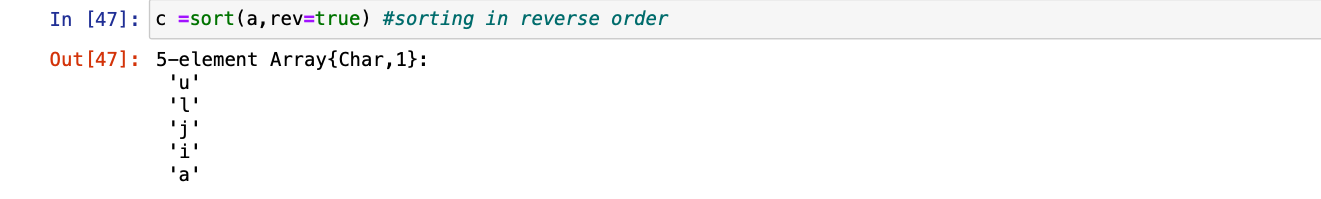My Personal Notes arrow_drop_up
Recommended Articles
Page :